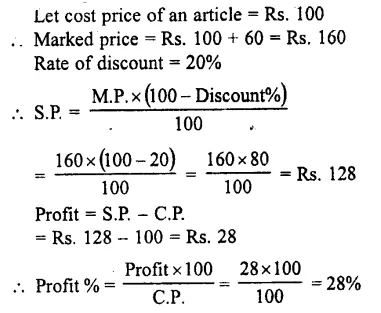## Selina Publishers Concise Mathematics Class 7 ICSE Solutions Chapter 9 Profit, Loss and Discount

Selina Publishers Concise Mathematics Class 7 ICSE Solutions Chapter 9 Profit, Loss and Discount

### Profit, Loss and Discount Exercise 9A – Selina Concise Mathematics Class 7 ICSE Solutions

Question 1.
Find the gain or loss percent, if
(i) C.P. = Rs. 200 and S.P.: = Rs. 224
(ii) C.P. = Rs. 450 and S.P. = Rs. 400
(iii) C.P. = Rs. 550 and gain = Rs . 22
(iv) CP. = Rs. 216 and loss = Rs. 72
(v) S.P. = Rs. 500 and loss : = Rs. 100
(vi) S.P. = Rs. 12 and profit = Rs. 4
(vii) C.P. = Rs. 5 and gain = 60 P

Solution:Question 2.
Find the selling price, if:
(i) C.P. = Rs. 500 and gain = 25%
(ii) C.P. = Rs. 60 and loss = 12 1/2%
(iii) C.P. = Rs. 150 and loss = 20%
(iv) C.P. = Rs. 80 and gain = 2.5%
Solution: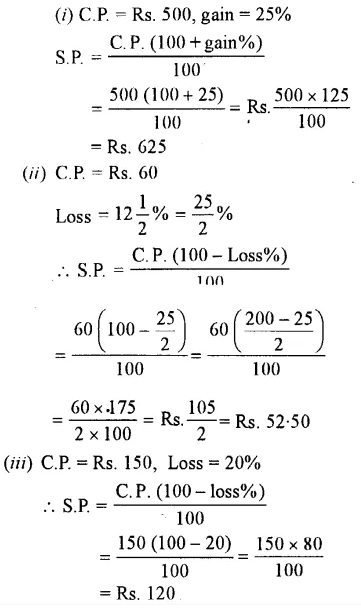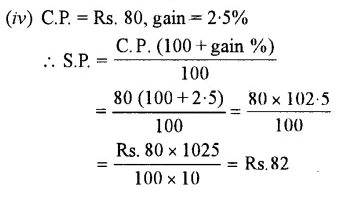Question 3.
Rohit bought a tape-recorder for Rs. 1,500 and sold it for Rs. 1,800. Calculate his profit or loss percent.
Solution:Question 4.
An article bought for Rs. 350 is sold at a profit of 20%. Find its selling price.
Solution:Question 5.
An old machine is bought for Rs. 1,400 and is sold at a loss of 15%. Find its selling price.
Solution: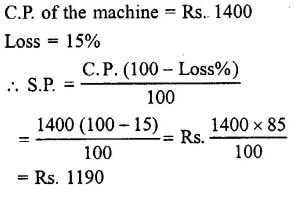Question 6.
Oranges are bought at 5 for Rs. 10 and sold at 6 for Rs. 15. Find profit or loss as percent.
Solution: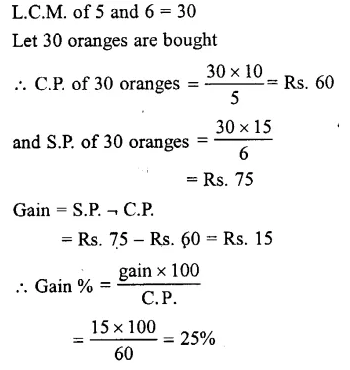Question 7.
A certain number of articles are bought at 3 for Rs. 150 and all of them are sold at 4 for Rs. 180. Find the loss or gain as percent.
Solution:Question 8.
A vendor bought 120 sweets at 20 p each. In his house, 18 were consumed and he sold the remaining at 30 p each. Find his profit or loss as percent.
Solution:Question 9.
The cost price of an article is Rs. 1,200 and selling price is $$\frac { 5 }{ 4 }$$ times of its cost price. Find:
(i) selling price of the article
(ii) profit or loss as percent.
Solution: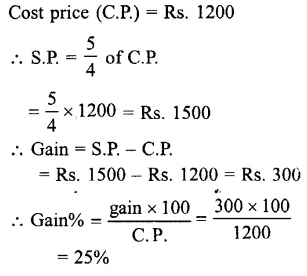Question 10.
The selling price of an article is Rs. 1,200 and cost price is $$\frac { 5 }{ 4 }$$ times of its selling price,
find :
(i) cost price of the article ;
(ii) profit or loss as percent.
Solution: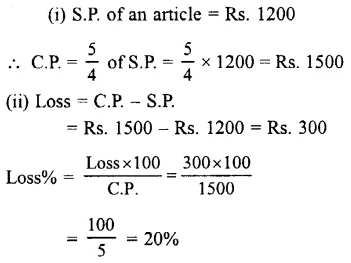### Profit, Loss and Discount Exercise 9B – Selina Concise Mathematics Class 7 ICSE Solutions

Question 1.
Find the cost price, if:
(i) S.P. = Rs. 21 and gain = 5%
(ii) S.P. = Rs. 22 and loss = 12%
(iii) S.P. = Rs. 340 and gain = Rs. 20
(iv) S.P. = Rs. 200 and loss = Rs. 50
(v) S.P. = Re. 1 and loss = 5 p.
Solution:Question 2.
By selling an article for Rs. 810, a loss of 10 percent is suffered. Find its cost price.
Solution:Question 3.
By selling a scooter for Rs. 9,200, a man gains 15%. Find the cost price of the scooter.
Solution:Question 4.
On selling an article for Rs. 2,640, a profit of 10 percent is made. Find
(i) cost price of the article
(ii) new selling price of it, in order to gain 15%
Solution: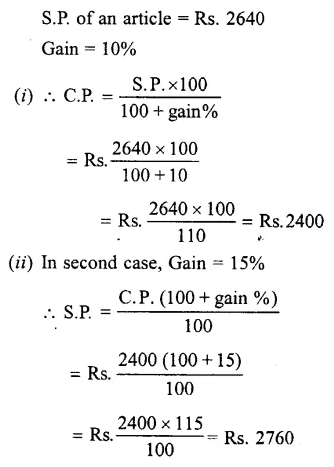Question 5.
A T.V. set is sold for Rs. 6800 at a loss of 15%. Find
(i)cost price of the T.V. set.
(ii)new selling price of it, in order to gain 12%
Solution: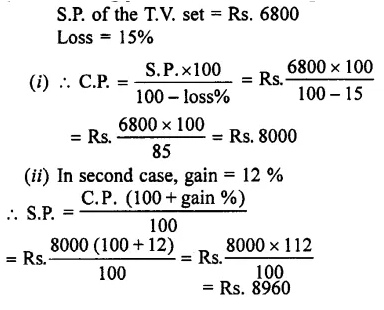Question 6.
A fruit seller bought mangoes at Rs. 90 per dozen and sold them at a loss of 8 percent. How much will a customer pay for.
(i) one mango
(ii) 40 mangoes
Solution:Question 7.
By selling two transistors for Rs. 00 each, a shopkeeper gains 20 percent on one transistor and loses 20 percent on the other.
Find :
(i) C.P. of each transistor

(ii) total C.P. and total S.P. of both the transistors
(iii) profit or loss percent on the whole.
Solution: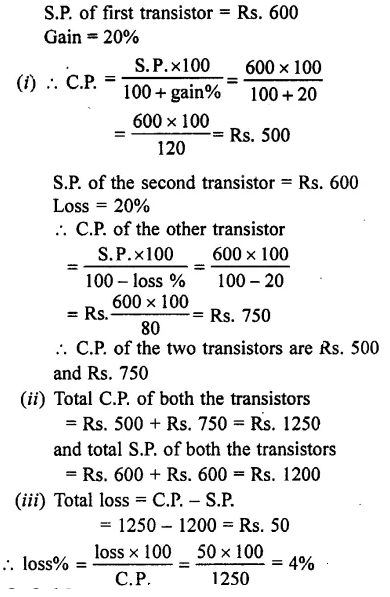Question 8.
Mangoes are bought at 20 for Rs. 60. If
they are sold at 33$$\frac { 1 }{ 3 }$$ percent profit.
Find:

(i) selling price of each mango.
(ii) S.P. of 8 mangoes.
Solution: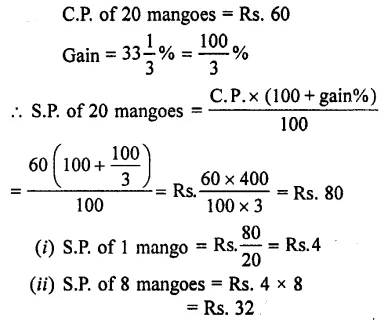Question 9.
Find the cost price of an article, which is sold for Rs. 4050 at a loss of 10%. Also, find the new selling price of the article which must give a profit of 8%.
Solution: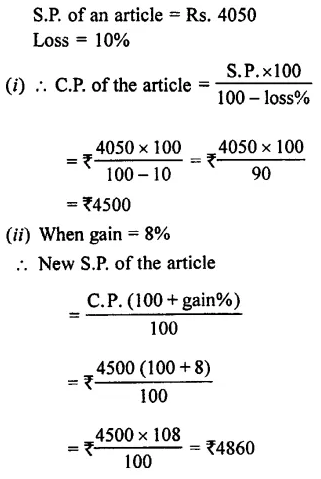Question 10.
By selling an article for ₹825, a man loses $$\frac { 1 }{ 3 }$$ equal to j of its selling price.
Find :

(i) the cost price of the article,
(ii) the profit percent or the loss percent made, if the same article is sold for ₹1265.
Solution: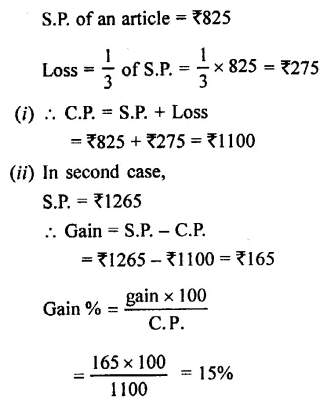Question 11.
Find the loss or gain as percent, if the C.P. of 10 articles, all of the same kind, is equal to S.P. of 8 articles.
Solution: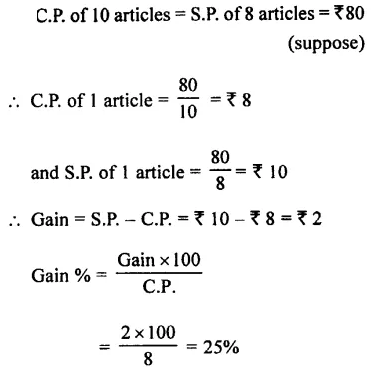Question 12.
Find the loss or gain as percent, if the C.P. of 8 articles, all of the same kind, is equal to S.P. of 10 articles.
Solution: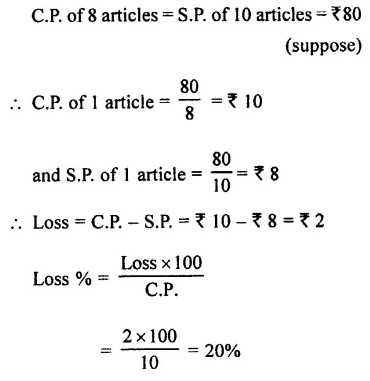Question 13.
The cost price of an article is 96% of its selling price. Find the loss or the gain as percent on the whole.
Solution: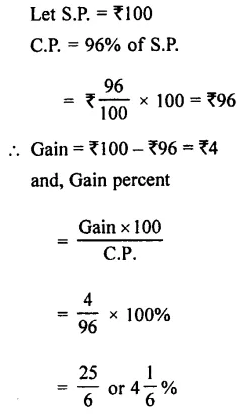Question 14.
The selling price of an article is 96% of its cost price. Find the loss or the gain as percent on the whole.
Solution: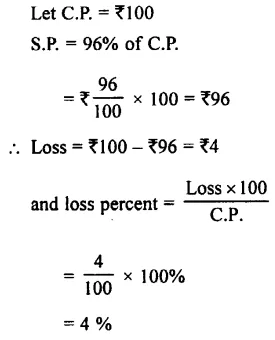Question 15.
Hundred oranges are bought for ₹350 and all of them are sold at the rate of ₹48 per dozen. Find the profit percent or loss percent made.
Solution: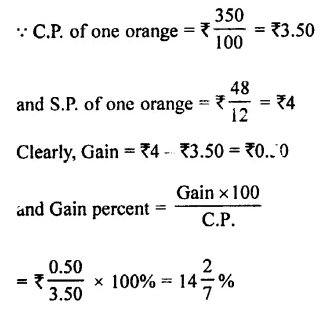Question 16.
Oranges are bought at 100 for ?80 and all of them are sold at ₹80 for ₹100. Find the loss or gain as percent in this transaction.
Solution: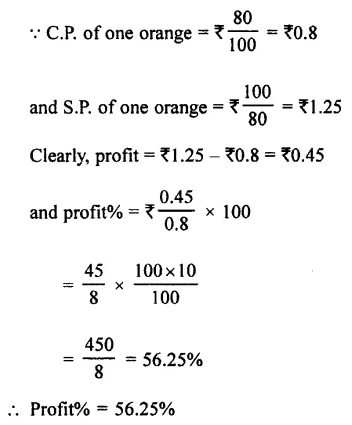Question 17.
An article is bought for ₹5,700 and ₹1,300 is spent on its repairing, transportion, etc. For how much should this article be sold in order to gain 20% on the whole.
Solution:
<### Profit, Loss and Discount Exercise 9C – Selina Concise Mathematics Class 7 ICSE Solutions

Question 1.
A machine is marked at ₹5000 and is sold at a discount of 10%. Find the selling price of the machine.
Solution: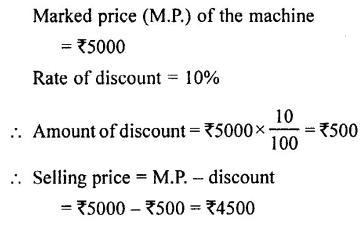Question 2.
shopkeeper marked a dinner set for ₹1000. He sold it at ₹900, what percent discount did he give ?
Solution: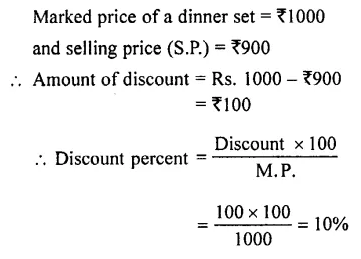Question 3.
A pair of shoes marked at ₹320, are sold at a discount of 15 percent.
Find :
(i) discount
(ii) selling price of the shoes.
Solution: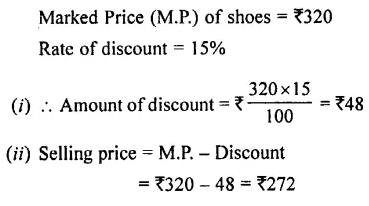Question 4.
The list price of an article is ₹450 and it is sold for ₹360.
Find :
(i) discount
(ii) discount percent
Solution: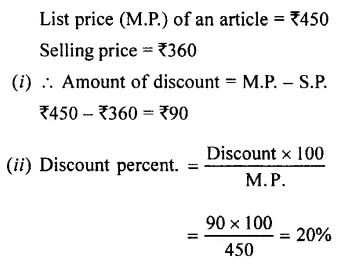Question 5.
A shopkeeper buys an article for₹300. He increases its price by 20% and then gives 10% discount on the new price. Find:
(i) the new price (marked price) of the article.
(ii) the discount given by the shopkeeper.
(iii) the selling price.
(iv) profit percent made by the shopkeeper.
Solution: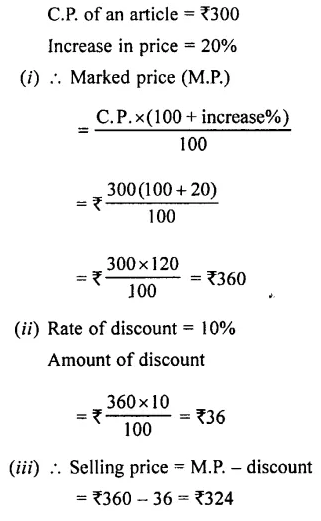Question 6.
A car is marked at Rs. 50,000. The dealer gives 5% discount on first Rs. 20,000 and 2% discount on the remaining Rs. 30,000.
Find :
(i) the total discount.
(ii) the price charged by the dealer.
Solution:Question 7.
A dealer buys a T.V. set for Rs. 2500. He marks it at Rs. 3,200 and then gives a discount of 10% on it.
Find :
(i) the selling price of the T.V. set
(ii) the profit percent made by the dealer.
Solution: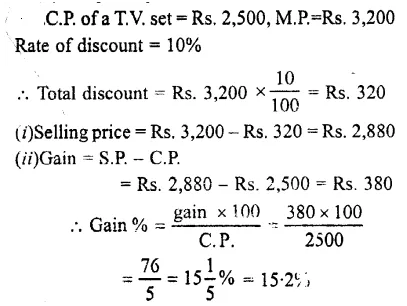Question 8.
A sells his goods at 15% discount. Find the price of an article which is sold for Rs. 680.
Solution: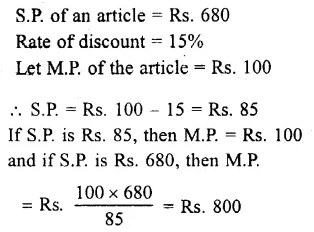Question 9.
A shopkeeper allows 20% discount on the marked price of his articles. Find the marked price of an article for which he charges Rs. 560.
Solution: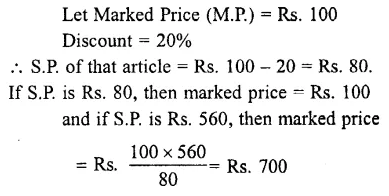Question 10.
An article is bought for Rs. 1,200 and Rs. 100 is spent on its transportation, etc.
Find :
(i) the total C.P. of the article.
(ii) the selling price of it in order to gain 20% on the whole.
Solution: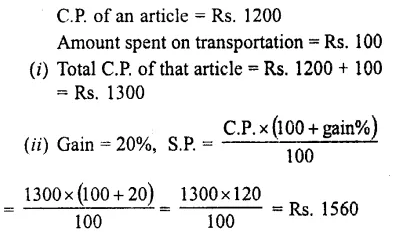Question 11.
40 pens are bought at 4 for Rs. 50 and all of them are sold at 5 for Rs. 80
Find :
(i) C.P. of one pen.
(ii) S/P. of one pen.
(iii) Profit made by selling one pen.
(iv) Profit percent made by selling one pen.
(v) C.P. of 40 pens
(vi) S.P. of 40 pens.
(vii) Profit made by selling 40 pens.
(viii) Profit percent made by selling 40 pens. Are the results of parts (iv) and (viii) same? What conclusion do you draw from the above result ?
Solution: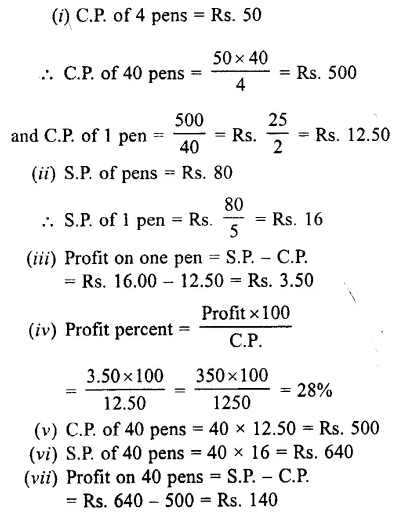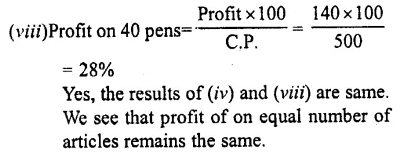Question 12.
The C.P. of 5 identical articles is equal to S.P. of 4 articles. Calculate the profit percent or loss percent made if all the articles bought are sold.
Solution: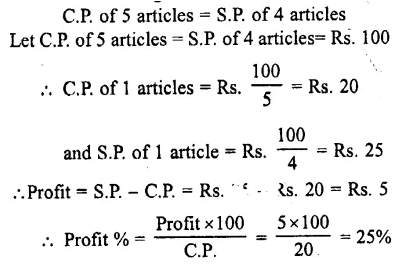Question 13.
The C.P. of 8 pens is same as S.P. of 10 pens. Calculate the profit or loss percent made, if all the pens bought are considered to be sold
Solution: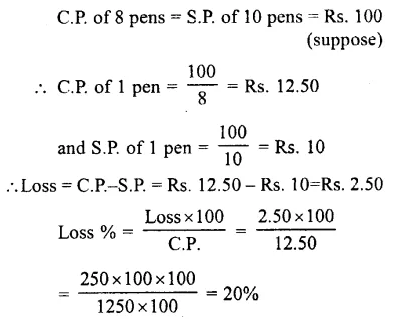Question 14.
A certain number of articles are bought at Rs. 450 per dozen and all of them are sold at a profit of 20%. Find the S.P. of:
(i) one article
(ii)seven articles.
Solution: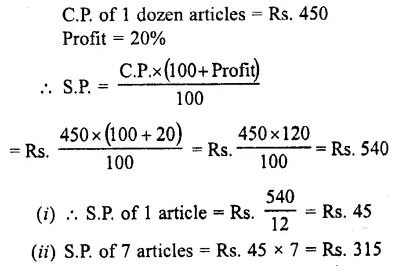Question 15.
An article is marked 60% above the cost price and sold at 20% discount. Find the profit percent made.
Solution: